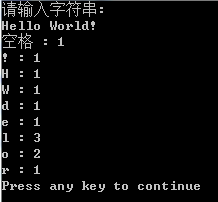每天一个小程序（13）——统计字符个数

#include <stdio.h>
#include <algorithm>

#define NUM 100

struct Data
{
char ch;
int count;
}data[NUM];

void print(char str,int count)//打印至屏幕，主要是为了显示“空格”
{
if(str == 32)
printf("%s : %d\n","空格",count);
else
printf("%c : %d\n",str,count);
}
void count(char str[],int n)
{
char *temp = str;
std::sort(temp,temp+n);//进行排序
char a1,a2;
int count = 0;
int num = 0;
a1 = temp[num++];
count++;
while(num <= n)
{
a2 = temp[num++];
if(a1 == a2)//碰到相同的 count加1
{
count++;
}
else//碰到不同的，输出字符和count，并记录新的字符
{
print(a1,count);
a1 = a2;
count = 1;
}
}
}

void main()
{
char str[NUM];
printf("请输入字符串:\n");
gets(str);
int len = strlen(str);
count(str,len);
}

PS：这个程序是区分大小写的